# Equations and Problem Solving Using Algebra to solve

• Slides: 13Equations and Problem Solving Using Algebra to solve Word Problems: Counting Problems and Mixture ProblemsDefinition CONSECUTIVE INTEGERS Integers that differ by one. The integers 50 and 51 are consecutive and so are -10 and -9 The sum of 3 consecutive integers is 147. Find the integers. Let n = the first integer Then n+1 = the second integer And n+2 = the third integer Let n = 48 Then n+1 = 49 And n+2 = 50 9/29/2020 n + n+1 + n+2 n+n+1+n+2 3 n + 3 3 n n www. numberbender. com =n 147 = 144 = 48 2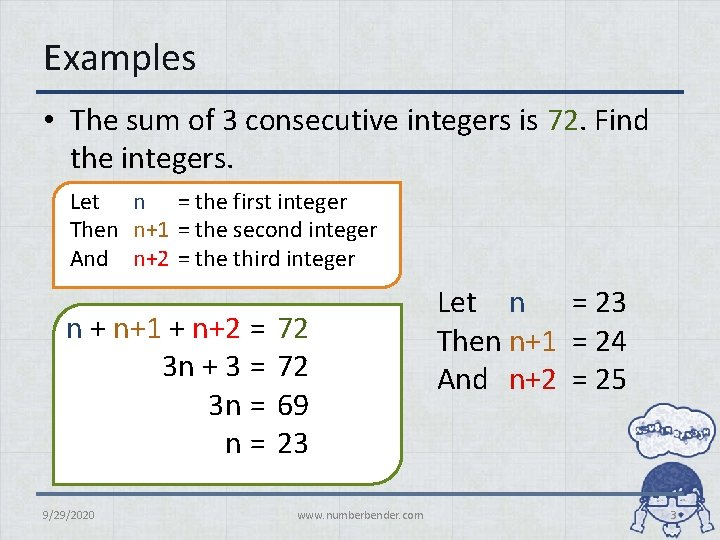Examples • The sum of 3 consecutive integers is 72. Find the integers. Let n = the first integer Then n+1 = the second integer And n+2 = the third integer n + n+1 + n+2 = 3 n + 3 = 3 n = n= 9/29/2020 72 72 69 23 www. numberbender. com Let n = 23 Then n+1 = 24 And n+2 = 25 3Examples • The sum of 3 consecutive integers is 915. Find the integers. Let n = the first integer Then n+1 = the second integer And n+2 = the third integer n + n+1 + n+2 = 3 n + 3 = 3 n = n= 9/29/2020 915 912 304 www. numberbender. com Let n = 304 Then n+1 = 305 And n+2 = 306 4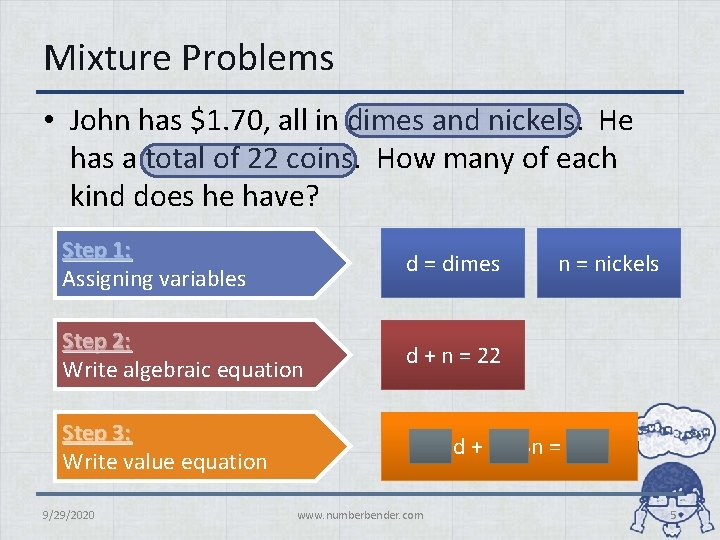Mixture Problems • John has \$1. 70, all in dimes and nickels. He has a total of 22 coins. How many of each kind does he have? Step 1: Assigning variables d = dimes Step 2: Write algebraic equation d + n = 22 Step 3: Write value equation 0. 10 d + 0. 05 n = 1. 70 9/29/2020 www. numberbender. com n = nickels 5Mixture Problems • John has \$1. 70, all in dimes and nickels. He has a total of 22 coins. How many of each kind does he have? Step 4: Solve the mixture problem (100)(0. 10 d + 0. 05 n) = 1. 70 (100) 10 d + 5 n = 170 (100) ( d + n)= 22 (100) 10 d + 10 n = 220 9/29/2020 www. numberbender. com 6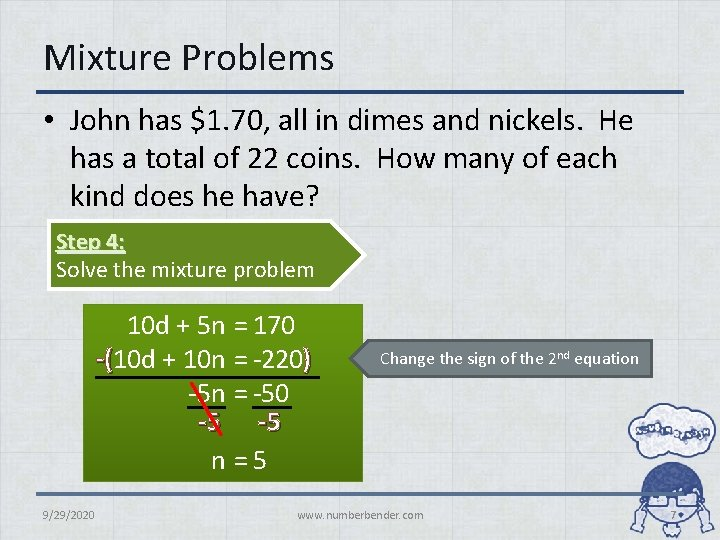Mixture Problems • John has \$1. 70, all in dimes and nickels. He has a total of 22 coins. How many of each kind does he have? Step 4: Solve the mixture problem 10 d + 5 n -(10 d + 10 n -5 n 9/29/2020 = 170 = -220) = -50 -5 =5 Change the sign of the 2 nd equation www. numberbender. com 7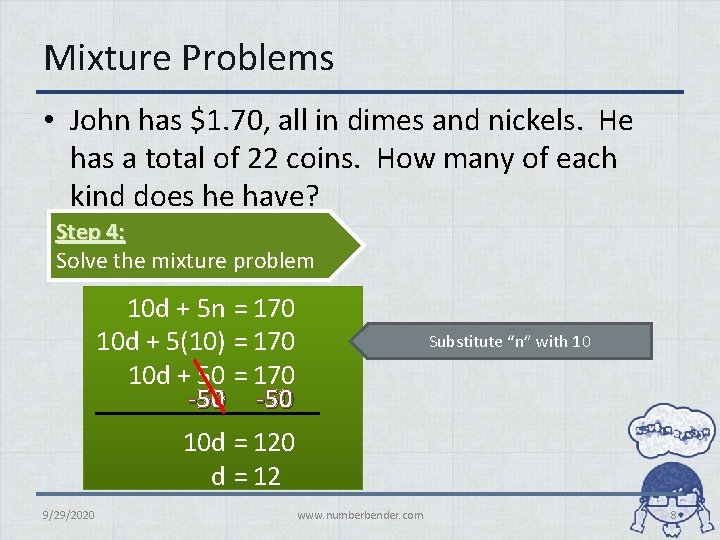Mixture Problems • John has \$1. 70, all in dimes and nickels. He has a total of 22 coins. How many of each kind does he have? Step 4: Solve the mixture problem 10 d + 5 n = 170 10 d + 5(10) = 170 10 d + 50 = 170 -50 Substitute “n” with 10 10 d = 120 d = 12 9/29/2020 www. numberbender. com 8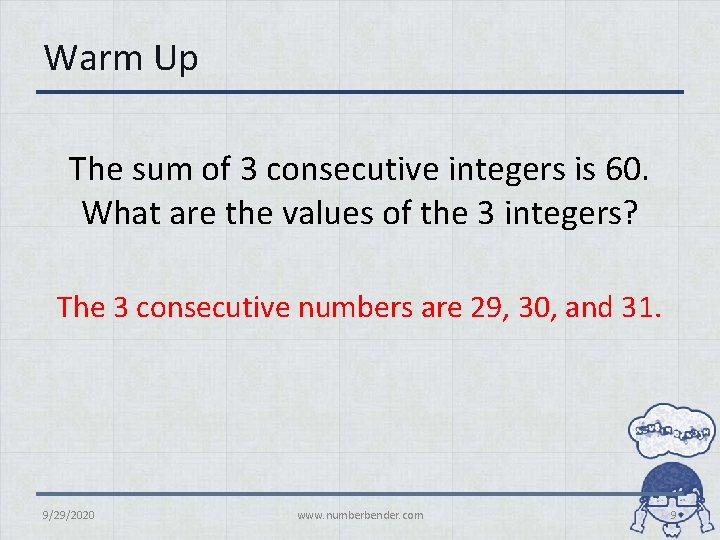Warm Up The sum of 3 consecutive integers is 60. What are the values of the 3 integers? The 3 consecutive numbers are 29, 30, and 31. 9/29/2020 www. numberbender. com 9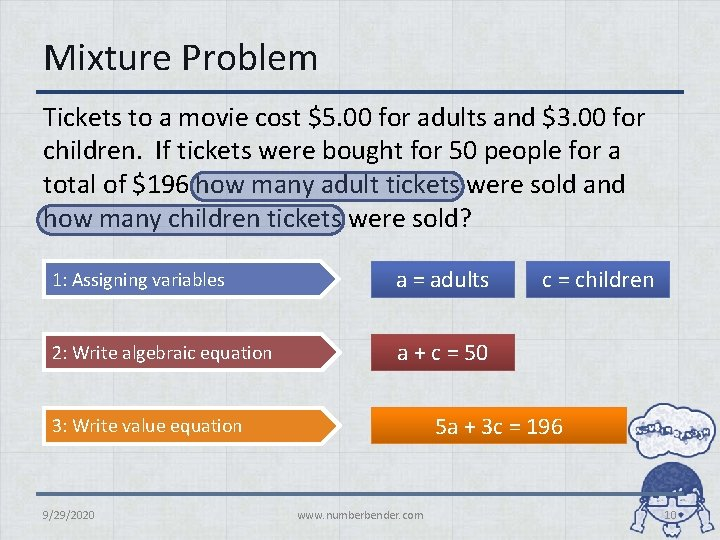Mixture Problem Tickets to a movie cost \$5. 00 for adults and \$3. 00 for children. If tickets were bought for 50 people for a total of \$196 how many adult tickets were sold and how many children tickets were sold? 1: Assigning variables a = adults 2: Write algebraic equation a + c = 50 5 a + 3 c = 196 3: Write value equation 9/29/2020 c = children www. numberbender. com 10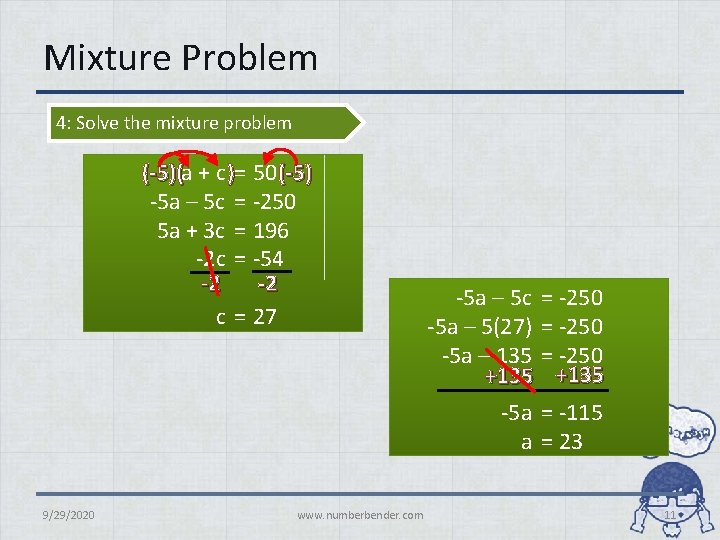Mixture Problem 4: Solve the mixture problem (-5)(a + c )= 50 (-5) -5 a – 5 c = -250 5 a + 3 c = 196 -2 c = -54 -2 -2 c = 27 9/29/2020 www. numberbender. com -5 a – 5 c = -250 -5 a – 5(27) = -250 -5 a – 135 = -250 +135 -5 a = -115 a = 23 11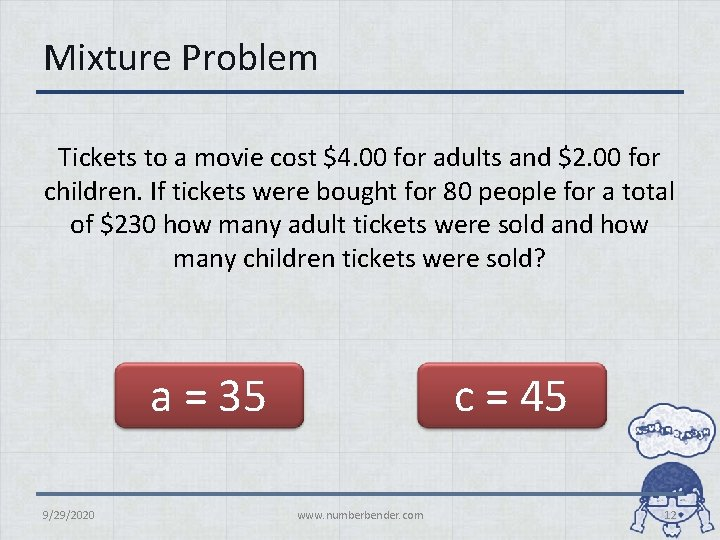Mixture Problem Tickets to a movie cost \$4. 00 for adults and \$2. 00 for children. If tickets were bought for 80 people for a total of \$230 how many adult tickets were sold and how many children tickets were sold? a = 35 9/29/2020 c = 45 www. numberbender. com 12Homework Amy has 32 coins consisting of dimes and quarters. If Amy has a total of \$5 in her pocket, how many of each coin are there? dimes = 20 9/29/2020 quarters = 12 www. numberbender. com 13